Opposite Puzzles

Fill in the boxes with the letters to the right so that two words of equal length are formed which are opposites of each other. Letters in connected boxes are the same, and are only given once to the right. Each puzzle has a unique solution.

 1.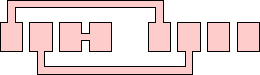H L P S U 2.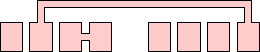E L M O R S 3.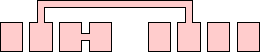A F I L P S 4.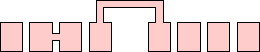C H I O R P 5.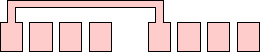A D K N S U W 6.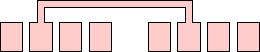D F L O S T U 7.A D E H R S Y 8.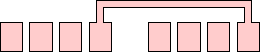A E H L O T V 9.A E G I K T V 10.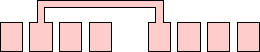C E G L T U Y 11.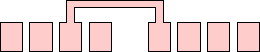A F L O S T W 12.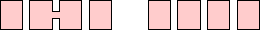A C L M O R W 13.E D G I L O V 14.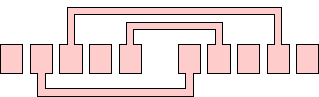A B E L O V W 15.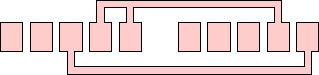B C E L R S U 16.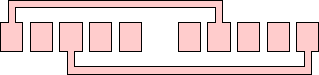G H I N O R T W 17.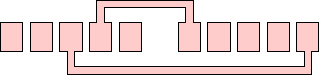A E F H L R S T 18.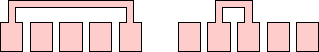E G H I L O S T 19.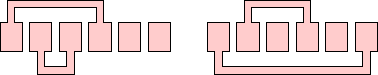A C D E F K N T 20.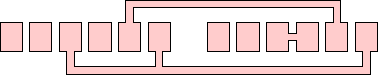A E F L M O R T 21.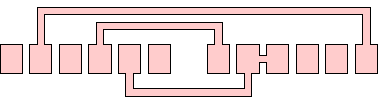A D E I P R T V

The solutions can be found here.